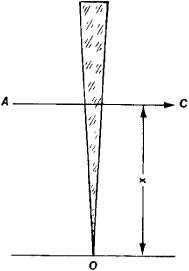# Photometer Wedge

The following article is from The Great Soviet Encyclopedia (1979). It might be outdated or ideologically biased.

## Photometer Wedge

a device for attenuating a light flux, used in photometry. It is a wedge made from an achromatic (neutral gray) substance whose absorption coefficient is independent of the wavelength of the light (special glass or a gelatin film containing colloidal graphite or silver). The attenuation of the light flux in any portion of a photometer wedge is determined by its optical density D= log (φ0/φ), where φ0/φ is the ratio of the light fluxes incident on the wedge and passing through it. The optical density may change along the wedge either continuously, increasing proportional to its thickness l(continuous wedge), or in steps of a particular size (step wedge).

Photometer wedges are characterized by a constant k, which in the case of the continuous wedge is equal to the difference between the optical densities of any of its points separated by a unit length and in the case of the step wedge is equal to the difference between the optical densities of two adjacent fields. The linear relationship of l and D to the distance x between the origin O of the wedge and the portion AC under consideration (see Figure 1) makes it possible to apply to it a uniform scale, which is calibrated with respect to the constant k.Figure 1

The movement of a wedge, which is indicated on the scale, makes possible variation of its transmission coefficient π = (φ/φ0 = (1 - p)2 X 10-kx, where p is the reflection coefficient for each surface of the wedge. Photometer wedges are made in both linear and circular forms.

### REFERENCE

Shishlovskii, A. A. Prikladnaia fizicheskaia optika. Moscow, 1961.